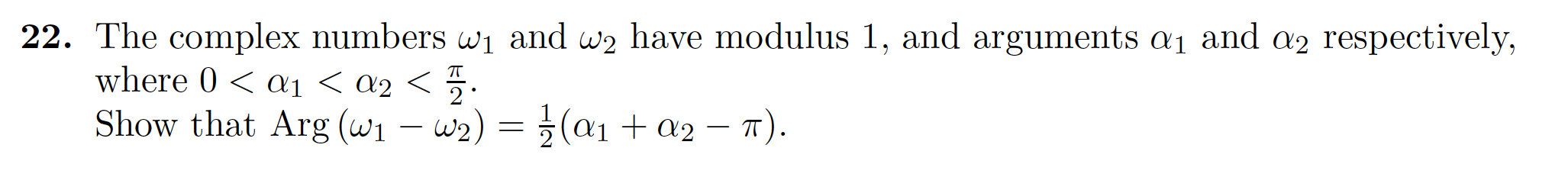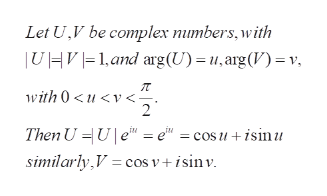# 22. The complex numbers wi and w2 have modulus 1, and arguments ai and a2 respectively,where 0< a1 < a2 <Show that Arg (w1 -w2)= (a1+a2-T).T2

Question
17 views

[1E q22]

Question is attached, Thanks ?help_outlineImage Transcriptionclose22. The complex numbers wi and w2 have modulus 1, and arguments ai and a2 respectively, where 0< a1 < a2 < Show that Arg (w1 -w2)= (a1+a2-T). T 2 fullscreen
check_circle

Step 1

To prove the equality of the two angles , under the given conditions

Step 2

Writing down U, V in the usual form ...help_outlineImage TranscriptioncloseLet U,V be complex numbers,with UHV1, and arg (U) = u,arg(V) = v. with 0

### Want to see the full answer?

See Solution

#### Want to see this answer and more?

Solutions are written by subject experts who are available 24/7. Questions are typically answered within 1 hour.*

See Solution
*Response times may vary by subject and question.
Tagged in

### Math